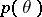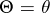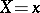# MediaWiki:Sidebar

A conditional probability distribution of a random variable, to be contrasted with its unconditional or a priori distribution.

Let <html>be a random parameter with an a priori density, letbe a random result of observations and letbe the conditional density ofwhen; then the a posteriori distribution offor a given</html>, according to the Bayes formula, has the density

<html></html>

todo

How to Cite This Entry:
Sidebar. Encyclopedia of Mathematics. URL: http://www.encyclopediaofmath.org/index.php?title=Sidebar&oldid=2998